• Instant Asset Write Off

# \$150,000 Instant Asset Write-Off

The \$150,000 Instant Asset Write-Off provides businesses with an asset write-off. Assets must cost less than the instant asset write-off threshold and be purchased and used in the year that the write-off is claimed.

What do you get?
An asset write-off of up to \$150,000.

Who is this for?

# Overview

The \$150,000 Instant Asset Write-Off provides businesses with an asset write-off of up to \$150,000 for assets costing less than the instant asset write-off threshold which are purchased and used in the year that the write-off is claimed.

# What are the eligibility criteria?

To be eligible, you must:

• have an aggregated turnover of less than \$500 million

• Assets must:

• cost less than the instant asset write-off threshold
• be purchased and used in the year the write-off is claimed

• # How do you apply?

There is no application required. The write-off is applied when you lodge your tax for the relevant year.

# Hot Offers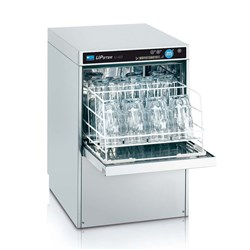\$2,679.23 ex GST (EACH)
Increase value Decrease value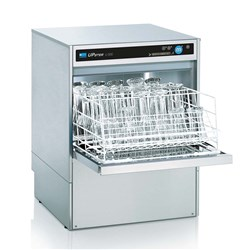\$3,570.56 ex GST (EACH)
Increase value Decrease value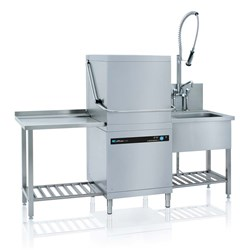\$4,528.32 ex GST (EACH)
Increase value Decrease value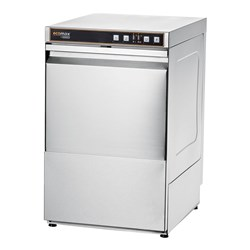\$2,445.00 ex GST (EACH)
Increase value Decrease value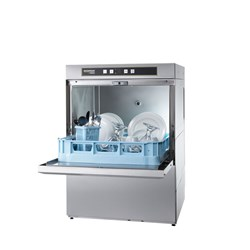\$3,545.00 ex GST (EACH)
Increase value Decrease value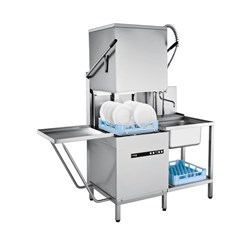\$4,855.00 ex GST (EACH)
Increase value Decrease value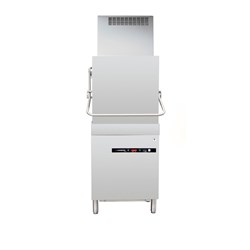\$9,999.00 ex GST (EACH)
Increase value Decrease value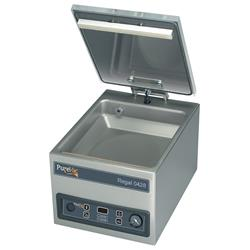\$2,625.00 ex GST (EACH)
Increase value Decrease value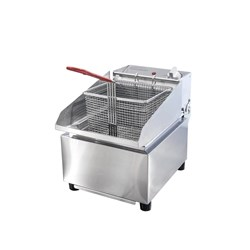\$375.00 ex GST (EACH)
Increase value Decrease value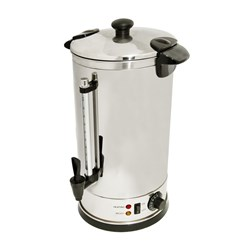\$175.00 ex GST (EACH)
Increase value Decrease value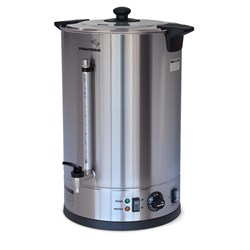\$195.00 ex GST (EACH)
Increase value Decrease value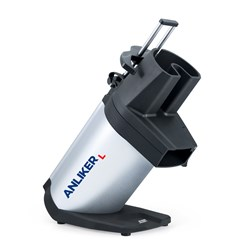\$3,599.00 ex GST (EACH)
Increase value Decrease value
\$2,178.45 ex GST (EACH)
Increase value Decrease value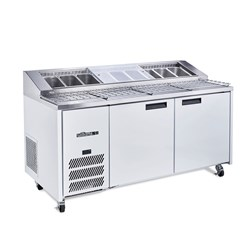\$5,055.00 ex GST (EACH)
Increase value Decrease value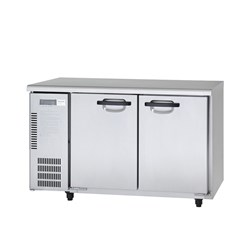\$2,375.00 ex GST (EACH)
Increase value Decrease value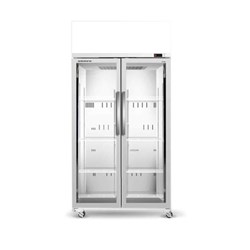\$2,160.00 ex GST (EACH)
Increase value Decrease value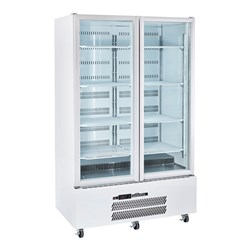\$3,299.00 ex GST (EACH)
Increase value Decrease value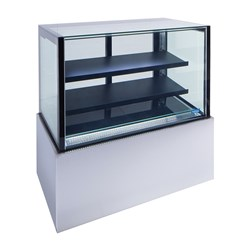\$3,055.00 ex GST (EACH)
Increase value Decrease value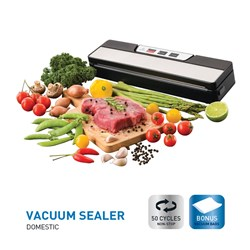\$99.95 ex GST (EACH)
Increase value Decrease value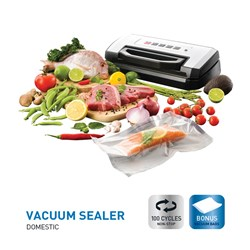\$199.95 ex GST (EACH)
Increase value Decrease value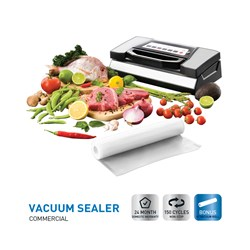\$299.95 ex GST (EACH)
Increase value Decrease value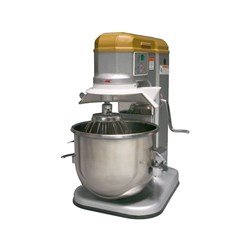\$1,055.00 ex GST (EACH)
Increase value Decrease value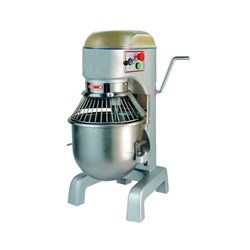\$2,290.00 ex GST (EACH)
Increase value Decrease value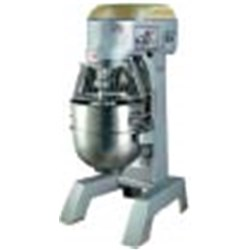\$4,950.00 ex GST (EACH)
Increase value Decrease value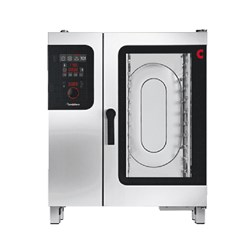\$19,900.00 ex GST (EACH)
Increase value Decrease value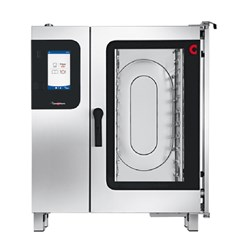\$23,900.00 ex GST (EACH)
Increase value Decrease value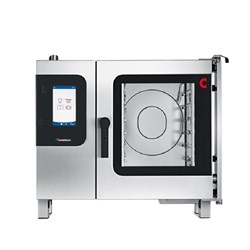\$17,690.00 ex GST (EACH)
Increase value Decrease value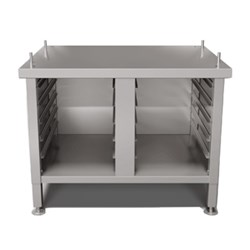\$995.00 ex GST (EACH)
Increase value Decrease value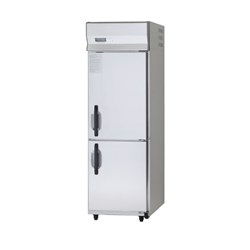\$2,850.00 ex GST (EACH)
Increase value Decrease value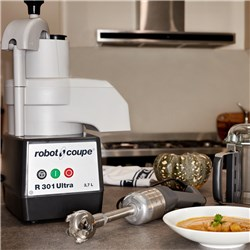\$2,645.00 ex GST (EACH)
Increase value Decrease value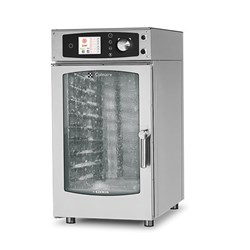\$11,995.00 ex GST (EACH)
Increase value Decrease value
\$2,775.85 ex GST (EACH)
Increase value Decrease value
\$13,666.81 ex GST (EACH)
Increase value Decrease value
\$10,490.93 ex GST (EACH)
Increase value Decrease value
\$13,801.41 ex GST (EACH)
Increase value Decrease value
\$14,241.54 ex GST (EACH)
Increase value Decrease value
\$2,475.20 ex GST (EACH)
Increase value Decrease value
\$919.17 ex GST (EACH)
Increase value Decrease value
\$1,199.00 ex GST (EACH)
Increase value Decrease value
\$2,253.00 ex GST (EACH)
Increase value Decrease value
\$4,450.59 ex GST (EACH)
Increase value Decrease value
\$4,736.00 ex GST (EACH)
Increase value Decrease value
\$2,526.00 ex GST (EACH)
Increase value Decrease value
\$1,995.00 ex GST (EACH)
Increase value Decrease value
\$3,310.00 ex GST (EACH)
Increase value Decrease value
\$885.21 ex GST (EACH)
Increase value Decrease value
\$1,848.85 ex GST (EACH)
Increase value Decrease value
\$1,127.85 ex GST (EACH)
Increase value Decrease value
\$946.55 ex GST (EACH)
Increase value Decrease value
\$566.00 ex GST (EACH)
Increase value Decrease value
\$666.40 ex GST (EACH)
Increase value Decrease value
\$2,625.00 ex GST (EACH)
Increase value Decrease value
\$879.80 ex GST (EACH)
Increase value Decrease value
\$1,160.70 ex GST (EACH)
Increase value Decrease value
\$2,090.00 ex GST (EACH)
Increase value Decrease value
\$3,225.00 ex GST (EACH)
Increase value Decrease value